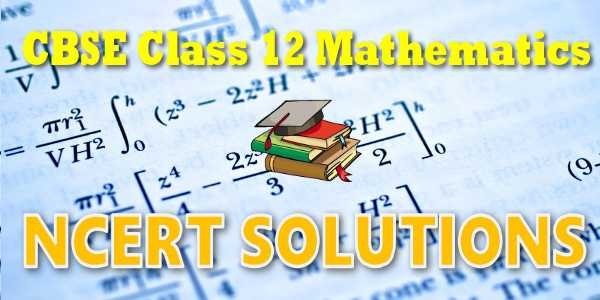No products in the cart.

# CBSE - Class 12 - Mathematics - Probability - NCERT Solutions

## NCERT Solutions for Mathematics Probability### Student Subscription

##### Unlock the exclusive content designed for the toppers

We will update content for this category shortly. Please visit this category after few days or subscribe to our newsletter by email for latest updates

# NCERT solutions for class 12 Mathematics## NCERT Class 12 Mathematics Chapter-wise Solutions

### Maths Part I

1. Chapter 1 – Relations and Functions
2. Chapter 2 – Inverse Trigonometric Functions
3. Chapter 3 – Matrices
4. Chapter 4 – Determinants
5. Chapter 5 – Continuity and Differentiability
6. Chapter 6 – Application of Derivatives

### Maths Part II

1. Chapter 7 – Integrals
2. Chapter 8 – Application of Integrals
3. Chapter 9 – Differential Equations
4. Chapter 10 – Vector Algebra
5. Chapter 11 – Three Dimensional Geometry
6. Chapter 12 – Linear Programming
7. Chapter 13 – Probability

## CBSE class 12th Mathematics have two books. Each book has chapters and topics.

1. NCERT Mathematics Book Class 12 Part-1
2. NCERT Mathematics Book Class 12 Part-2

Here is the list of topics covered under each chapter of class 12 Mathematics NCERT text book.

### Relations and Functions

• 1.1 Introduction
• 1.2Types of Relations
• 1.3Types of Functions
• 1.4Composition of Functions and Invertible Function
• 1.4Binary Operations

### 2. Inverse Trigonometric Functions

• 2.1 Introduction
• 2.2 Basic Concepts
• 2.3 Properties of Inverse Trigonometric Functions

### 3. Matrices

• 3.1 Introduction
• 3.2 Matrix
• 3.3 Types of Matrices
• 3.4 Operations on Matrices
• 3.5 Transpose of a Matrix
• 3.6 Symmetric and Skew Symmetric Matrices
• 3.7 Elementary Operation (Transformation) of a Matrix
• 8 Invertible Matrices

### 4. Determinants

• 4.1 Introduction
• 4.2 Determinant
• 4.3 Properties of Determinants
• 4.4 Area of a Triangle
• 4.5 Minors and Cofactors
• 4.6 Adjoint and Inverse of a Matrix
• 4.7 Applications of Determinants and Matrices

### 5. Continuity and Differentiability

• 5.1 Introduction
• 5.2 Continuity
• 5.3 Differentiability
• 5.4 Exponential and Logarithmic Functions
• 5.5 Logarithmic Differentiation
• 5.6 Derivatives of Functions in Parametric Forms
• 5.7 Second Order Derivative
• 5.8 Mean Value Theorem

### 6. Application of Derivatives

• 6.1 Introduction
• 6.2 Rate of Change of Quantities
• 6.3 Increasing and Decreasing Functions
• 6.4 Tangents and Normals
• 6.5 Approximations
• 6.6 Maxima and Minima

### 7.Integrals

• 7.1 Introduction
• 7.2 Integration as an Inverse Process of Differentiation
• 7.3 Methods of Integration
• 7.4 Integrals of some Particular Functions
• 7.5 Integration by Partial Fractions
• 7.6 Integration by Parts
• 7.7 Definite Integral
• 7.8 Fundamental Theorem of Calculus
• 7.9 Evaluation of Definite Integrals by Substitution
• 7.10 Some Properties of Definite Integrals

### 8. Application of Integrals

• 8.1 Introduction
• 8.2 Area under Simple Curves

### 9.Differential Equations

• 9.1 Introduction
• 9.2 Basic Concepts

### 10  Vector Algebra

• 10.1 Introduction
• 10.2 Some Basic Concepts
• 10.3 Types of Vectors
• 10.5 Multiplication of a Vector by a Scalar
• 10.6 Product of Two Vectors

### 12.Linear Programming

• 12.1 Introduction
• 12.2 Linear Programming Problem and its Mathematical Formula
• 12.3 Different Types of Linear Programming Problems

### 13. Probability

• 13.1 Introduction
• 13.2 Conditional Probability
• 13.3 Multiplication Theorem on Probability
• 13.4 Independent Events
• 13.5 Bayes' Theorem
• 13.6 Random Variables and its Probability Distributions
• 13.7 Bernoulli Trials and Binomial Distribution

## NCERT Solutions Chapter 13 Probability

In earlier Classes, we have studied the probability as a measure of uncertainty of events in a random experiment. We discussed the axiomatic approach formulated by Russian Mathematician, A.N. Kolmogorov (1903-1987) and treated probability as a function of outcomes of the experiment. We have also established equivalence between the axiomatic theory and the classical theory of probability in case of equally likely outcomes. On the basis of this relationship, we obtained probabilities of events associated with discrete sample spaces. We have also studied the addition rule of probability. In this chapter, we shall discuss the important concept of conditional probability of an event given that another event has occurred, which will be helpful in understanding the Bayes' theorem, multiplication rule of
probability and independence of events. We shall also learn an important concept of random variable and its probability distribution and also the mean and variance of a probability distribution. In the last section of the chapter, we shall study an important discrete probability distribution called Binomial distribution. Throughout this chapter, we shall take up the experiments having equally likely outcomes, unless stated otherwise.

## NCERT Solutions for Class 12th Mathematics

NCERT Solutions Class 12 Mathematics PDF (Download) Free from myCBSEguide app and myCBSEguide website. Ncert solution class 12 Mathematics includes text book solutions from both part 1 and part 2. NCERT Solutions for CBSE Class 12 Mathematics have total 13 chapters. Class 12 Mathematics ncert Solutions in pdf for free Download are given in this website. Ncert Mathematics class 12 solutions PDF and Mathematics ncert class 12 PDF solutions with latest modifications and as per the latest CBSE syllabus are only available in myCBSEguide.

## NCERT Solutions for Class 3## myCBSEguide

Trusted by 1 Crore+ Students

#### Question Paper Creator

• Create papers in minutes
• Print with your name & Logo
• 5 Lakhs+ Questions
• Solutions Included
• Based on CBSE Syllabus
• Best fit for Schools & Tutors## Test Generator

Create papers at ₹10/- per paper## CUET Mock Tests

75,000+ questions to practice only on myCBSEguide app